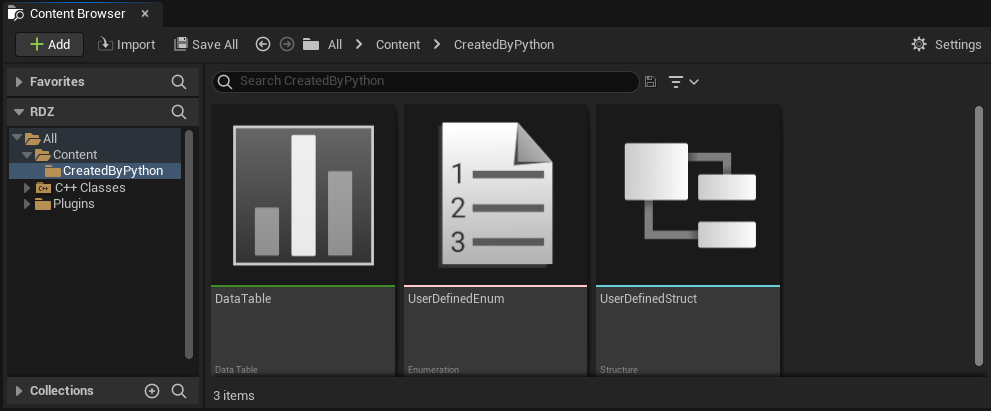TAPython has extended lots of editor functions in PythonEditorLibs. In the latest version 1.0.5, TAPython add 40+ Editor API for use the User Defined Enum, User Defined Struct and DataTable. Now we can create, query and modify the User Defined Enum/Struct/DataTable with python.

Make it as simple as possible, but not simpler.

In short, we can use Python to do almost everything you did manually in the editor with them. Download latest TAPython here

Below are the Cheat Sheets:

## User Defined Enum¶

### Create User Defined Enum¶

The code below will create a User Defined Enum asset at "/Content/CreatedByPython/IAmAEnum", with Unreal Engine's built-in function. In factor, other assets can be created using this method as well.

``````    asset_tools = unreal.AssetToolsHelpers.get_asset_tools()
new_enum = asset_tools.create_asset("IAmAEnum", "/Game/CreatedByPython", unreal.UserDefinedEnum, unreal.EnumFactory())
``````

Then we need add enum items to the Enum.

``````    items = ["A", "BB", "CCC", "DDDD", "EEEEE"]
unreal.PythonEnumLib.set_enum_items(self.current_enum, items)
``````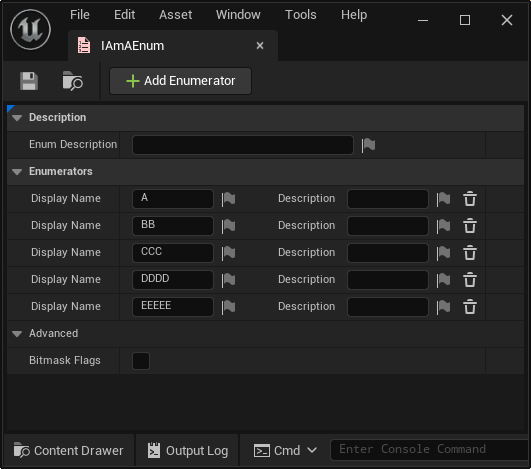The Variable self.current_enum is the Enum we created in above. We can also load it from the package path.

``````    self.current_enum = unreal.load_asset("/Game/CreatedByPython/IAmAEnum")
``````

### Modify User Defined Enum¶

We can modify a specified item with a new name.

We can set the "name" with PythonEnumLib.set_display_name. The User Defined Enum has Two names, one is "Display Name" which used in UI, and another is the "Raw Name", the real name of enum item.

``````    # The enum item at index 3 will be: "iAmItem_3"
unreal.PythonEnumLib.set_display_name(self.current_enum, 3, "iAmItem_{}".format(3))
``````

#### Modfiy Enum's Description¶

The description of Enum is the description of Enum. It's an EditorProperty of UObject, so we can edit it with SetEditorProperty

``````    # set the description of enum
self.current_enum.set_editor_property("enum_description", "Enum Description setted by Python")
``````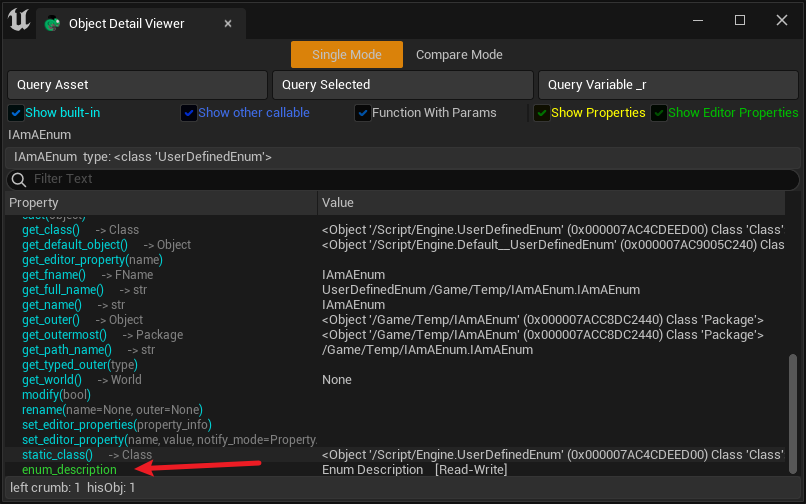#### Modfiy Enum item's Description¶

Each Enum item also has an individual description. We can modify it with PythonEnumLib.set_description_by_index

``````    # we can iter each enum item, and set
for i in range(unreal.PythonEnumLib.get_enum_len(self.current_enum)):
unreal.PythonEnumLib.set_description_by_index(self.current_enum, i, f"item description {i}")
``````

#### Set BitFlags Flag¶

``````    unreal.PythonEnumLib.set_bitflags_type(self.current_enum, True)
``````

#### Moves the Enum item¶

We can move the enum iten to target index, other items will be shifting as needed. For example, with enum items: [A, B, C, D, E], moving index 1 to index 3 results in [A, C, D, B, E].

``````    unreal.PythonEnumLib.move_enum_item(self.current_enum, 1, 3)
``````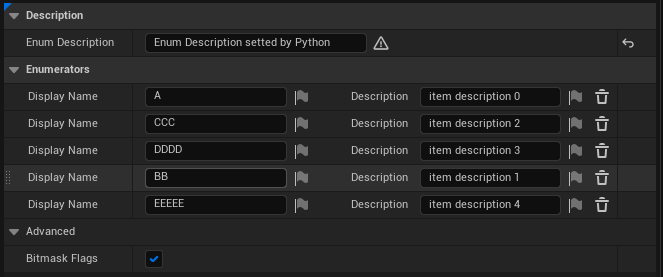## User Defined Struct¶

As an important Data Assets, User Defined Structs also need to be able to be created, queried, and modified.

### Create User Defined Struct¶

The way of create a User Defined Struct is similar to creating Enum.

``````    asset_tools = unreal.AssetToolsHelpers.get_asset_tools()
result = asset_tools.create_asset("IAMStruct", "/Game/CreateByPython", unreal.UserDefinedStruct, unreal.StructureFactory())
``````

### Modify User Defined Struct¶

Add variable is a little more complex than adding an item to the Enum. As a variable, it can be a bool, float, struct, enum, interface, object or even a soft object class. And the data "container" type also could be: single, array, set or map.

The function doc of PythonStructLib.add_variable:

``` add_variable(...) X.add_variable(struct, category, sub_category, sub_category_object, container_type_value, is_reference=False) -> bool Add a new variable to specified User Defineded Strcut

``````Args:
struct (UserDefinedStruct):         The User Defineded Strcut you want to modify.
category (Name):                    The Category of the new Variable
sub_category (Name):                The SubCategory of the new Variable
sub_category_object (Object):       The SubCategoryObject of the new Variable
container_type_value (int32):       Container type. 0: single, 1: array, 2: set. Use add_directory_variable if you want add a map variable
is_reference (bool): Whether e new Variable passed as reference

Returns:
bool: True if the new variable has been added
``````

```

Let's look some examples:

• Add a bool variable:``````    unreal.PythonStructLib.add_variable(self.current_struct, "bool", "", None, 0, False)
``````
• Add a int variable: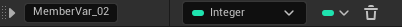``````    unreal.PythonStructLib.add_variable(self.current_struct, "int", "", None, 0, False)
``````
• Add a int64(long) variable: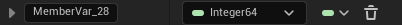``````    unreal.PythonStructLib.add_variable(self.current_struct, "int64", "", None, 0, False)
``````
• Add a float Array variable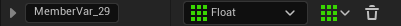``````    unreal.PythonStructLib.add_variable(self.current_struct, "real", "double", None, container_type_value=1, is_reference=False)
``````
• Add a Name(FName) Set variable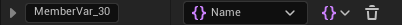``````    unreal.PythonStructLib.add_variable(self.current_struct, "string", "", None, container_type_value=2, False)
``````
• Add a Vector variable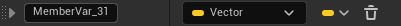``````    unreal.PythonStructLib.add_variable(self.current_struct, "struct", "", unreal.Vector.static_struct(), 0, False)
``````
• Add a Transform variable``````    unreal.PythonStructLib.add_variable(self.current_struct, "struct", "", unreal.Transform.static_struct(), 0, False)
``````

In fact, Vector, Rotator, Transform are all "struct".

• Add another Struct variable``````    unreal.PythonStructLib.add_directory_variable(self.current_struct, 'struct', '', unreal.AbcMaterialSettings.static_struct(), 0)
``````
• Add a Interface variable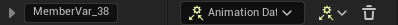``````   unreal.PythonStructLib.add_variable(self.current_struct, 'interface', '', unreal.AnimationDataController.static_class(), 0, False)
``````
• Add the User Denfind Enum that create above, which also created by python code.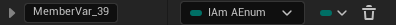``````    unreal.PythonStructLib.add_variable(_r, "byte", "", unreal.load_asset('/Game/CreatedByPython/IAmAEnum'), 0, False)
``````
• Add the User Denfind Enum which was defined in Engine Content: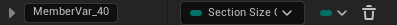``````    unreal.PythonStructLib.add_variable(self.current_struct, 'byte', '', unreal.load_asset('/Landmass/Landscape/BP/Enums/SectionSizeOptions'), 0)
``````
• Add a actor variable: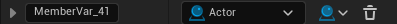``````    # Add Actor Reference
unreal.PythonStructLib.add_variable(self.current_struct, "object", "", unreal.Actor.static_class(), 0, False)
``````
• Add a actor class variable: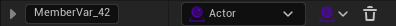``````    # Object Class Reference
unreal.PythonStructLib.add_variable(self.current_struct, "class", "", unreal.Actor.static_class(), 0, False)
``````
• Add a soft actor variable: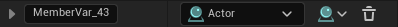``````     # Object Reference
unreal.PythonStructLib.add_variable(self.current_struct, "softobject", "", unreal.Actor.static_class(), 0, False)
``````
• Add a actor soft class variable: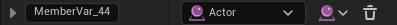``````    unreal.PythonStructLib.add_variable(self.current_struct, "softclass", "", unreal.Actor.static_class(), 0, False)
``````

### Note¶

• Not all datatype can be a Set or a Map variable. Only the "Hashable" type could.
• The boolean value, "True" is hashable, but it can't be a Set or a Map variable.

In PythonStructLib.add_variable we use the param: container_type_value to specify the variable is "Single", "Array" or a "Set" variable. If we want to add a Map(dict) variable, we can use unreal.PythonStructLib.add_directory_variable

The code below will add a key-value map variable in the struct, the type of the key is actor soft class, and the value type is "string".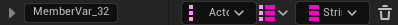``````    unreal.PythonStructLib.add_directory_variable(_r, "softclass", "", unreal.Actor.static_class(), False, "string", "", None)
``````

### And how could I know the a variable's "Category", "SubCategory" or "sub_category_object"?¶

We could add the target variable by hand in the editor, then log the detail with [PythonStructLib.log_var_desc]https://www.tacolor.xyz/pages/PythonEditorLib/PythonStructLib.html##log-var-desc)

``````    unreal.PythonStructLib.log_var_desc(self.current_struct)
``````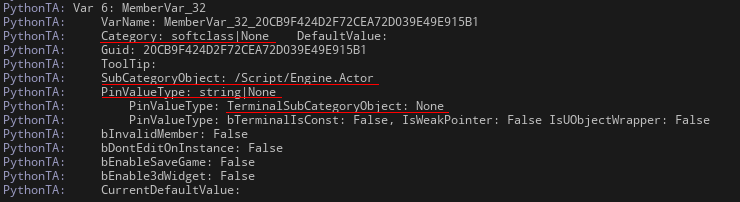And we can see: "Category: softclass|None" are the values of "Category", "SubCategory"; SubCategoryObject is "/Script/Engine.Actor";
"PinValueType: string|None" are the values of "Terminal_Category", "Terminal_Sub_Category" which used for a Map container type value. TerminalSubCategoryObject is None.

And also we can get the var desc as a dict. PythonStructLib.get_variable_description

``````    desc = unreal.PythonStructLib.get_variable_description(self.current_struct, var_name)
``````

## DataTable¶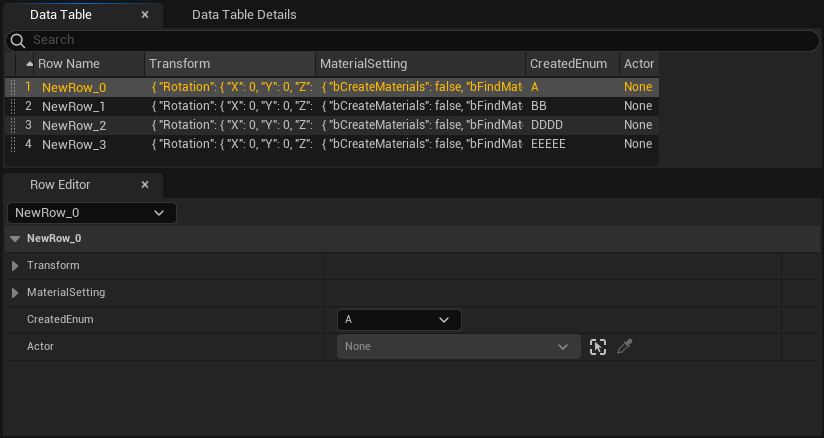### Create DataTable¶

Creating DataTable is also an easy job using Python. The only difference with Creating Struct is that the struct is set to DataTableFactory. For example, the following example directly uses the "IAMStruct" created in Python above.

``````    asset_tools = unreal.AssetToolsHelpers.get_asset_tools()
factory = unreal.DataTableFactory()
result = asset_tools.create_asset("IAmADataTable", "/Game/CreatedByPython", unreal.DataTable, factory)
``````

### Modify DataTable¶

#### Add a row¶

We can add a new row with the code below.

``````    unreal.PythonDataTableLib.add_row(self.current_datatable, row_name)
``````

The "row_name" need to be unique in the datatable. So we can get and check it with the existing names.

``````    exists_names = unreal.PythonDataTableLib.get_row_names(self.current_datatable)
bUnique = name not in exists_names
``````

#### Remove a row¶

``````    unreal.PythonDataTableLib.remove_row(self.current_datatable, row_name)
``````

#### Rename a row¶

A rename function is also useful.

``````    unreal.PythonDataTableLib.rename_row(self.current_datatable, row_name, new_row_name)
``````

#### Get Content of DataTable¶

There are several ways to get the content of the datatable with PythonDataTableLib.

We can get it as a JSON object.

``````    unreal.PythonDataTableLib.get_table_as_json(self.current_datatable)
``````

Or get it as a flatten array, and it's dimension(shape) can be get with get_shape

``````    unreal.PythonDataTableLib.get_flatten_data_table(self.current_datatable, include_header=False)`
row_count, column_count = unreal.PythonDataTableLib.get_shape(self.current_datatable)
``````

We can also get the cell content of datatable.

``````    # get value
current_value = unreal.PythonDataTableLib.get_property_as_string_at(self.current_datatable, row_index, column_index)
``````

#### Set the content of datatable¶

We can set every cell content of datatable with PythonDataTableLib.set_property_by_string_at

``````    # set value
unreal.PythonDataTableLib.set_property_by_string_at(self.current_datatable, row_index, column_index, new_value)
``````

This example will assign the static mesh which located in '/Game/Somewhere/YourMesh' to the datatable.

``````    # modify the static mesh reference in datatable at row 1 column 2
new_value = "StaticMesh'/Game/Somewhere/YourMesh.YourMesh_0'"
unreal.PythonDataTableLib.set_property_by_string_at(self.current_datatable, 1, 2, new_value)
``````

## Summary¶

Now, we can manipulate User Defined ENum, Struct, DataTable with Python in UE. The next step will be creating blueprint, and The final goal is to create the entire Unreal project in Python without any manual work? I hope so.

Share On